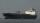# Bob and Bobek

At 10h 20min runs Bobek from hat at average speed 12 km/h. At what time must run out Bob at speed 18 km/h to catch him 9 kilometers from the hat?

Result

h = 10:35#### Solution:

12(t-(10+20/60))=9
18(t-h)=9

720t = 7980
18h-18t = -9

h = 12712 ≈ 10.583333
t = 13312 ≈ 11.083333

Calculated by our linear equations calculator.

Leave us a comment of example and its solution (i.e. if it is still somewhat unclear...):

Showing 0 comments:Be the first to comment!#### To solve this example are needed these knowledge from mathematics:

Do you have a linear equation or system of equations and looking for its solution? Or do you have quadratic equation?

## Next similar examples:

1. The shipThe ship went upstream speed of 20 km/h in relation to water. River flows at speed 10 km/h. After half an hour, he stopped and returned downstream to the starting point. How long it took way back when even if downstream speed of ship is 20 km/h in relation
2. Grandfather and grandmotherThe old mother is 5 years younger than the old father. Together they are 153 years old. How many years has each of them?
3. Ages 2A man's age is 4 times his son's age. After 5 years he will be just twice his son's age, find their ages.
4. Father and sonWhen I was 11, my father was 35 years old. Today, my father has three times more years than me. How old is she?
5. Vijuviju has 40 chickens and rabbits. If in all there are 90 legs. How many rabbits are there with viju??
6. TreesAlong the road were planted 250 trees of two types. Cherry for 60 CZK apiece and apple 50 CZK apiece. The entire plantation cost 12,800 CZK. How many was cherries and apples?
7. Spain vs USASpain lost to the US by 4 goals. In the match total fell 10 goals. How many goals gave the Spain and how the United States?
8. Belgium vs ItalyBelgium played a match with Italy and Belgium win by 2 goals. The match fell a total 6 goals. Determine the number of goals scored by Belgium and by Italy.
9. EquationSolve the equation: 1/2-2/8 = 1/10; Write the result as a decimal number.
10. Two trainsThere were 159 freight wagons on the railway station creating 2 trains. One had 15 more wagons than the other. How many wagons did each train have?
11. Football seasonDalibor and Adam together scored 97 goals in the season. Adam scored 9 goals more than Dalibor. How many goals scored each?
12. Football match 4In a football match with the Italy lost 3 goals with Germans. Totally fell 5 goals in the match. Determine the number of goals of Italy and Germany.
13. Two numbersWe have two numbers. Their sum is 140. One-fifth of the first number is equal to half the second number. Determine those unknown numbers.
14. Substitutionsolve equations by substitution: x+y= 11 y=5x-25
15. Family parcelsIn father will he divided the land so that the older son had three bigger part than younger son. Later elder son gave 2.5 ha field to younger and they had both the same. Determine the area of family parcel.
16. Boys and girlsThere are 48 children in the sports club, boys are 10 more than girls. How many girls go to the club?
17. SchoolsThree schools are attended by 678 pupils. To the first attend 21 students more and to the third 108 fewer students than to second school. How many students attend the schools?